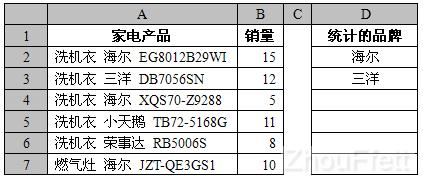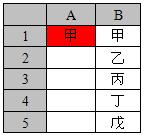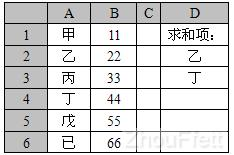_Excel公式教程

Value 必需。待检测的数值，可以是任意类型的单值。

（一）用于数据有效性。

=ISNUMBER(A1)

（二）用于条件格式。

=ISNUMBER(A1)

（三）用于函数中的逻辑值参数

=IF(AND(ISNUMBER(B1),ISNUMBER(D1),ISNUMBER(F1)),\"通过\",\"请完善\")

（一）用于条件格式。如果单元格包含指定的特征文本，则以特殊格式显示。

1、单元格A1如果包含文本“海尔”，则以高亮显示。可在A1条件格式设置以下公式及高亮格式：

=ISNUMBER(FIND(\"海尔\",A1))

=FIND(\"海尔\",A1)

2、单元格A1如果包含文本“海尔”或“美的”，则以粗体显示。可在A1条件格式设置以下公式及粗体格式：

=OR(ISNUMBER(FIND(\"海尔\",A1)),ISNUMBER(FIND(\"美的\",A1))) 或

=ISNUMBER(FIND(\"海尔\",A1))+ISNUMBER(FIND(\"美的\",A1))

3、把特征文本“海尔”“美的”“松下”放在单元格C1:C3，单元格A1如果包含这些文本，则以斜体显示。可在A1条件格式设置以下公式及斜体格式：

=OR(ISNUMBER(FIND(C\$1:C\$3,A1)))

4、把特征文本以数组常量的形式指定给一个名称，例如创建一个名称TZZ，其引用位置的公式为={\"海尔\",\"美的\",\"松下\"}。当单元格A1包含特征文本时以红色显示。可在A1条件格式设置以下公式及红色字体：

=OR(ISNUMBER(FIND(TZZ,A1)))

（二）对包含特征文本的行求和。如图：=SUMPRODUCT(ISNUMBER(FIND(\"海尔\",A2:A7))*B2:B7) 或

=SUMIF(A2:A7,\"*海尔*\",B2:B7)

=SUMPRODUCT(ISNUMBER(FIND({\"海尔\",\"三洋\"},A2:A7))*B2:B7) 或

=SUM(SUMIF(A2:A7,{\"*海尔*\",\"*三洋*\"},B2:B7)) 或

{=SUMPRODUCT(ISNUMBER(FIND(TRANSPOSE(D2:D3),A2:A7))*B2:B7)} 或

{=SUM(SUMIF(A2:A7,\"*\"&D2:D3&\"*\",B2:B7))}

（一）如果单元格的值在某列表中找得到，则以特殊格式显示。=ISNUMBER(MATCH(A1,\$B:\$B,))

=MATCH(A1,\$B:\$B,)

（二）对符合某些值的行求和。=SUMPRODUCT(ISNUMBER(MATCH(A1:A6,D:D,))*B1:B6)